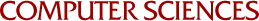## Computing Multiplicative Inverses in GP(p)

G. E. Collins
1968

Two familiar algorithms, the extended Euclidean algorithm and the Fermat algorithm (based on Fermat's theorem up 2 a(mod p)), are analyzed and compared as methods for computing multiplicative inverses in GF(p). Using Knuth's results on the average number of divisions in the Euclidean algorithm, it is shown that the average number of arithmetic operations required by the Fermat algorithm is nearly twice as large as the average number for the extended Euclidean algorithm. For each of the two algorithms, forward and backward versions are distinguished. It is shown that all numbers computed in the forward extended Euclidean algorithm are bounded by the larger of the two inputs, a property which was previously established by Kelisky for the backward version.